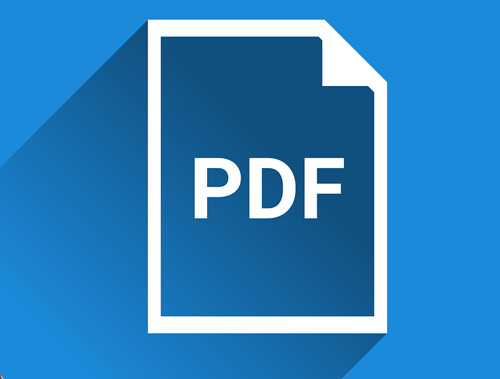Common Topics: pdf, text, data, import, file

Google has been indexing PDFs for many years and ranks them among web pages so it’s only logical to analyze your PDFs for optimization opportunities just like a web page. Obviously in general that is a much harder task given the file’s constraints, but we can start this process with the help of Python. I’m going to show you how easy it is to convert the PDF’s text into actual string text that we can then do any number of analyses on. We’re going to approach this using a crawl CSV from Screaming Frog (export PDF file data) because in many circumstances you may not even know if or how many PDFs are on your site.

## Requirements and Assumptions

• Python 3 is installed and basic Python syntax understood
• Crawl data with PDF files, or a CSV list of PDF URLs

Let’s start by installing PDFminer, a Python module that has the functions to convert the PDF text to string text we can use. If you are using Google Colab, put an exclamation mark before pip3 below.

`pip3 install pdfminer`

Now we import a number of modules.

• io: to handle writing text from a file to a variable
• pandas: adding PDF text data to a dataframe
• time: a small script delay
• pdfminer: will process the PDF
```from io import StringIO
import urllib.request
import pandas as pd
import time

from pdfminer.converter import TextConverter
from pdfminer.layout import LAParams
from pdfminer.pdfdocument import PDFDocument
from pdfminer.pdfinterp import PDFResourceManager, PDFPageInterpreter
from pdfminer.pdfpage import PDFPage
from pdfminer.pdfparser import PDFParser

Now we import the PDF crawl CSV file, address column only. Then we convert the address column to a list so we can iterate through it and process the PDFs.

```df_pdf = pd.read_csv('internal_pdf.csv')[['Address']]
print(list_pdf)```

We’ll now set a counter for the loop later when we store the PDF files locally. We will next create an empty dataframe that will eventually store the PDF text for each PDF file.

```counter = 0
df_pdf = pd.DataFrame([], columns=['URL','Text'])```

It’s time to process the PDF list! What need to do is download each of the PDFs locally and then process them. So the first variable set is filepath and we use the counter to help make each PDF name unique. You could also write some regex to strip everything but the file name in the URL but this was easier. Then we make the request using urllib.request.urlretrieve() as the common requests module can’t handle downloading files. The pdf variable contains the URL for the PDF and filepath is where the file will be saved and named locally. Lastly, we’ll sleep for 5 seconds to make sure the file is downloaded before we continue.

```for pdf in list_pdf:
filepath = "pdf" + str(counter) + ".pdf"
counter += 1

urllib.request.urlretrieve(pdf,filepath)
time.sleep(5)```

Now that we have downloaded a PDF file it’s time to process it which involves a bunch of functions from the PDFminer module we installed. In general, what is happening here is the file is opened and put into an object. Then the PDFminer parser/converter/interpreter process each page in the PDF and puts the text in the output_string variable.

```    output_string = StringIO()
with open(filepath, 'rb') as in_file:
parser = PDFParser(in_file)
doc = PDFDocument(parser)
rsrcmgr = PDFResourceManager()
device = TextConverter(rsrcmgr, output_string, laparams=LAParams())
interpreter = PDFPageInterpreter(rsrcmgr, device)
for page in PDFPage.create_pages(doc):
interpreter.process_page(page)```

Now that we have text in the output_string file object we can get the contents by using getvalue(). Returns are retained and converted to \n so we’ll search and replace those with a space.

```    text = output_string.getvalue()
text = text.replace('\n\n',' ')```

It’s time to load the PDF URL and its text into a dictionary object and finally add it to the dataframe. At this point, if you have more PDFs in your list the process starts again with the new PDF URL. When all is done, the dataframe will print out for you to see.

```    keyword_dict = {"URL":pdf,"Text":text}
df_pdf = df_pdf.append(keyword_dict, ignore_index=True)